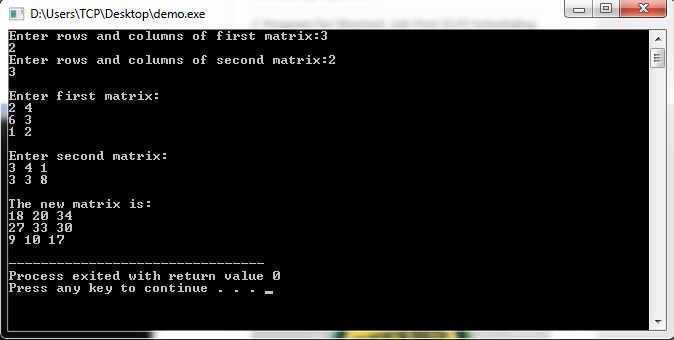# C++ Matrix Multiplication Program

Here you will get C++ matrix multiplication program.

What we are doing in this program.

Read number of rows and columns for two matrix.

Then check if matrix multiplication is possible or not.

If not possible then show a message to user otherwise multiply them. Finally display the result.

## C++ Matrix Multiplication Program

Output### 30 thoughts on “C++ Matrix Multiplication Program”

1.This somehow does not work/compile for me.
It throws out the follwing error:

No main found…simply generating .o files…
In file included from
/usr/lib/gcc/i386-redhat-linux/3.4.6/../../../../include/c++/3.4.6/backward/iostream.h:31,
from mat.cpp:1:
mat.cpp:2:18: conio.h: No such file or directory
mat.cpp:5: error: `main' must return `int'
mat.cpp:5: error: return type for `main' changed to `int'
mat.cpp: In function `int main(...)':mat.cpp:6: error: `clrscr' was not declared in this scope
mat.cpp:40: error: `getch' was not declared in this scope
mat.cpp:6: warning: unused variable 'clrscr'
mat.cpp:40: warning: unused variable 'getch'

1.By taking a look on the errors i concluded that you are using gcc on linux os. The above code is written in turbo c++ so it will not work in gcc.

1.This will work with gcc and clang and msvc as it doesn’t use any turbo c++ features
You need to call g++ in order to compile it as cpp code else this will throw an error every time you try to compile it.
CmdLine: g++ main.cpp -o main.out -std=c++11 -O3

2.Why do we use getch() before ending any program? What is the function of getch()?

1.Actually getch() is a function used to read character value without displaying it on the screen. When it is added at the end of the program, compiler waits and stops the screen for reading any character, as we press any key the program ends. I hope this will make you understand the use of getch().

2.I think u r new to c getch () is for inputting character value bt to keep output on the screen we hv to use getch other wise output will be terminate in just mili seccends

3.Getch function holds the programm output screen until any key is pressed.

3.why r u using c[i][j]=0

1.Jst to initialize destination array
U can skip c [i][j]=0

4.nice written but you must use //comments

5.why are we leave space btn cout<<[i][j]<<" ";

1.so that there are spaces in between the matrices and two numbers can be differentiated.
for ex:- 80 90 39 without space can be 809039. it will be difficult for us to know the numbers without spaces.

6.thanx …but correct the inverted commas

7.Why u r equating the rows and columns of two matrices ?!

1.Only then the multiplication is possible.

8.Your Website really helps me in passing my exams 😛 since I need to do ctrl + F on my notebook (real notebook) Hehhee.

1.Hey Shreekant, I am so happy to know that my website is helping you, keep visiting and recommend it to others 🙂

9.man that program isn’t helpful for me
may be it’s a wrong code

10.Hey the code gives the wrong answer when i input 1->9 for matrix 1 and 9->1 for matrix 2

11.hey…plz tell me the use of “k” in the program….

12.Hey I need some coding clarification ,Sorry to use this page in doing so .
I want to create a function with inputs of type vector and integer but the output should be a matrix .
{ If you are comfortable in matlab it has A = function_name(v,x) ; where x = 5;v = ones(5,1);A = zeros(5,4). }

I want to use the output matrix as input to another function .I’m new to c++ ,How could I do that ?
I tried a few methods ;but the function is of type void , which wont output a result ,and wont give me the freedom to do A = function_name(input1,input2) in c++.

13.14.using namespace std;

what does this line indicate

1.The built in C++ library routines are kept in the standard namespace. That includes stuff like cout, cin, string, vector, map, etc. Because these tools are used so commonly, it’s popular to add “using namespace std” at the top of your source code so that you won’t have to type the std:: prefix constantly.

15.thanks a lot

16.Piyush kumar dubey

is we can get the .cpp file which we direct copy and paste in our turbo c++ folder and the program will run automatically…

17.Write a C++ program for matrix multiplication. Multiplication function should notify if the order of the matrix is invalid, using exception.
Can you help me to solve this

18.19.